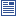Accessibility options:

# Simple Linear Regression resources

Show me all resources applicable to

### 03. Teach Yourself Worksheets (5)Simple Linear Regression - Introduction
A written introduction to Simple Linear Regression using the same examples as those used in the Video Tutorial available at this site. This resource was contributed by Dr Iain Weir of the University of the West of England.Simple Linear Regression (Worksheet 1)
Using an example on energy consumption, this teach yourself worksheet introduces simple linear regression and how to fit and interpret Linear Regression models using SPSS.Simple Linear Regression (Worksheet 2)
Using an example on calcium intake, this teach yourself worksheet introduces simple linear regression and how to fit and interpret Linear Regression models using SPSS. Note that the SPSS data set calcium.sav referred to in the worksheet is also available here.Simple Linear Regression (Worksheet 2) SPSS Data Set calcium.sav
This is a zip file containing the SPSS data set called calcium.sav for use with Simple Linear Regression (Worksheet 2) which considers an example on calcium intake. Note: please save this file to your PC before extracting its contents.Simple Linear Regression Assumptions and Predictions (Worksheet)
This teach yourself worksheet looks at the assumptions underlying simple linear regression, and how to obtain confidence intervals and prediction intervals using SPSS.